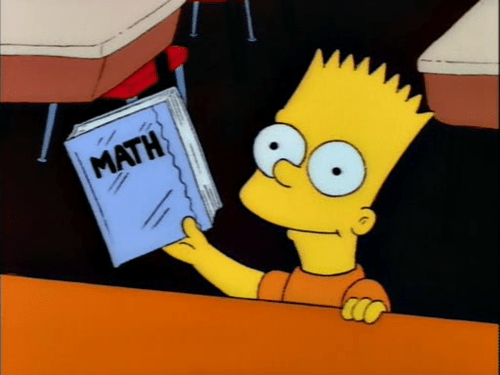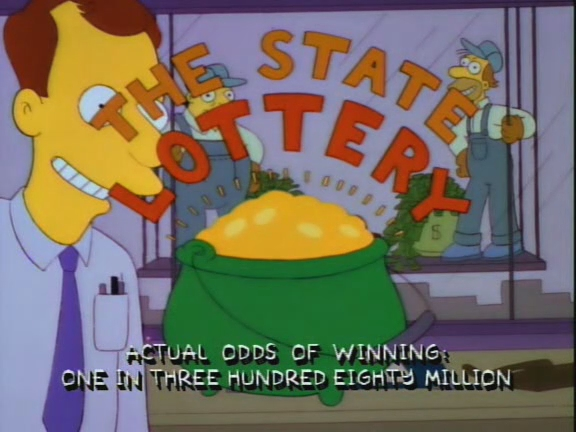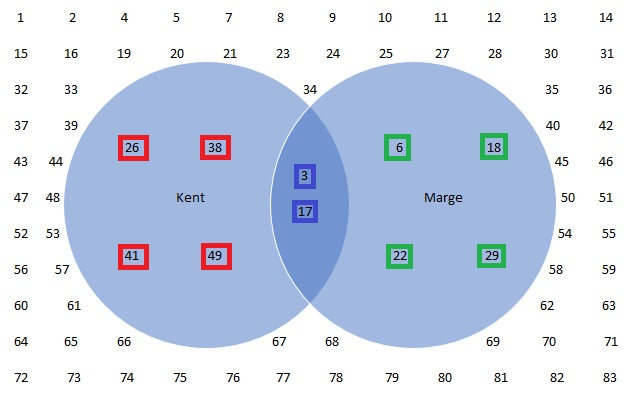# Super Safi’s Monday Morning Math Mayhem 33 – Statistics (Probabilities, Odds, Permutations, and Combinations)

Morning Mathematical Monsters & Maniacs!

(Today’s post is sponsored by the letter “M”)

Hi, I’m Super Safi and you may remember me from such stats and strategy posts as Kwik-E-Mart Farming and the advanced losing-to-win Superheroes battle strategy.

Over the past 600+ episodes, The Simpsons has taken us on an amazing mathematical journey involving fractions, probability, Fermat’s last theorem, and hundreds of other aspects from the wonderful world off mathematics.

And what better way to start your week, then by discussing math Monday morning?This week we continue our discussion of the lottery from last week while looking at probability, odds, permutations, and combinations.

In Dog of Death (Season 03, Episode 19), Springfield is “in the grip of lottery fever” with a \$130 million jackpot.The winning numbers of the \$130 million jackpot are 3, 17, 26, 38, 41, and 49 (which Kent Brockman wins). The following week, the numbers are 3, 6, 17, 18, 22 and 29 (which Marge would have won if the Simpsons family weren’t cutting corners to make ends meet). So we know that you must select 6 numbers.

However, in my Venn diagram depicting the lottery numbers, did you notice I went up to 83? There’s a reason for that.At the bottom of the lottery commercial, it says “Actual odds of winning: one in three hundred eighty millions”.

STATISTICS

First let’s start by exploring some basic definitions of a few mathematical terms:

Probability: A numerical description of how likely an event is to occur or how likely it is that a proposition is true. Probability is a number between 0 and 1, with 0 indicating impossibility and 1 indicating certainty. The higher the probability of an event, the more likely it is that the event will occur.

Odds: The relative probability an event will occur. Odds can be expressed in a few different manners, including the above example from The Simpsons in which it is stated how many times the outcome will happen (1) in how many tries (380,000,000).

Permutations and Combinations: The various ways numbers from a set can be selected, with the difference being that in permutations order matters and in combinations order does not matter. Lotteries are generally combinations, as the order the numbers are announced in does not matter; as long as you get all the numbers right.

Going back to the episode of the Simpsons, why did my Venn diagram go up to 83?

We know that you must select 6 numbers to win the lottery, we know that the odds of winning are 1 in 380,000,000. We also know Kent correctly selected 49, so there are at least 49 numbers to select from.

There are a few ways to calculate how many numbers are in the Springfield lottery. Let’s call the highest number you can select x.

The first time the lottery selects a number, you can have 6 out of x numbers on your way to selecting each one correctly. The next time, you can get 5 out of x – 1. The next time, 4 out of x – 2. Then 3 out of x – 3. Then 2 out of x – 4. And finally to get the last number correct, you have to get the 1 correct number out of x – 5. So…

(6/x) x (5/(x-1)) x (4/(x-2)) x (3/(x-3)) x (2/(x-4)) x (1/(x-5))   =   1/380,000,000

Solving for x gets you pretty darn close to 83.

(6/83) x (5/82) x (4/81) x (3/80) x (2/79) x (1/78)   =   1 / 377,447,148

Another way to calculate the odds of the lottery uses combinations. We know you must select 6 numbers out of x. To calculate combinations, we use our old friend from Math Mayhem 28, factorials. The notation to denote selecting 6 numbers out of x, is xC6.

xC6   =   x! / (6! x (x-6)!)   =   (x x (x-1) x (x-2)…3 x 2 x 1) / ((6 x 5 x 4 x 3 x 2 x 1) x ((x-6) x (x-5) x (x-4)…3 x 2 x 1))   =  (x x (x-1) x (x-2) x (x-3) x (x-4) x (x-5)) / 720   =   380,000,000

Solving for x to 1 in 380,000,000 also result in pretty darn close to 83.

83 x 82 x 81 x 80 x 79 x 78 / 720   =   377,447,148

Now that we’ve completed a look at probability, why not show your love for math and probability with your own Math Mayhem shirt or hoodie.

If you love math or enjoy reading these posts, don’t forget to stop by the Addicts Shop and check out all the paraphernalia, including the Math Mayhem shirts and hoodies.Were you familiar with Venn diagrams? What’s your favourite Venn diagram? Do you recall the episode featuring Venn diagrams from 20 years ago? Did you recall the episode with lottery numbers? Are you a dweek, dork, geek, nerd, or none of the above? Sound off in the comments below. You know we love hearing from you.

### 7 responses to “Super Safi’s Monday Morning Math Mayhem 33 – Statistics (Probabilities, Odds, Permutations, and Combinations)”

1.Alfred Chiu

Semi off topic but has anyone been getting the age verification and requires agreement in privacy and user info but that leads you off app which ends up refreshing the age confirmation. It’s an endless loop

2.karenskuality

In your first effort, explaining the odds, you have the expression and say “solving for x gets you pretty close to …”. But this is a SIXTH DEGREE EQUATION IN X, and you just gloss over the finding of all six roots (excluding some which might be negative or complex, I admit…I didn’t have the heart to find the roots, I’m resting for Christmas break!) and say “x=83 gets you pretty close”. Seems like there’s of “sweat equity” involved here? And it’s not just as simple as “x^6 and a constant”, lots of terms in between?Lol…nice post, good work here!

•NicoRox13

The denominator is x (x-1) (x-2) (x-3) (x-4) (x-5).

As x increases it increases and therefore the denominator increases

The approach Safi took was a numerical method. Kind of a proof by exhaustion.

Typically when they give odds for for lottery the round off to some number. In this case millions.

And yes, statistics was one of my majors.

3.hungryhomer

This is off topic and I posted this in last weeks open thread but got no reply. Hoping for better luck here.
I have a question about the task manager. So when you buy Old Tut and the Universal Panacea, Robo Burns has a task at the Panacea. So now when you use the task manager it automatically sends him there. Is there any way to get it to change it back to automatically send him on his task with Puppy Dog Smithers?

•Sandi

Store panaca, if not placed can’t do the task.

•Sandi

*Pancea

•hungryhomer

That stores old tut too